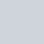Livio/ June 19, 2018/ Excel, Power Query/ 1 comments

## Replicate SUMPRODUCT in Power Query

Today I stumbled upon the M function List.Zip which I thought would provide a valid alternative to my previous post  about replicating SUMPRODUCT in Power Query.

According to Microsoft Power Query help: List.Zip takes a list of lists, and returns a list of lists combining items at the same position. This means that if you have 3 lists consisting of {{x1,x2,x3,x4,x5}, {y1,y2,y3,y4,y5}, {z1,z2,z3,z4,z5}}, List.Zip will turn it into {{x1,y1,z1},{x2,y2,z2},{x3,y3,z3},{x4,y4,z4},{x5,y5,z5}}. Pretty amazing and useful stuff.

Thanks to this, we can build another function which takes in a list of lists and performs the SUMPRODUCT:

PQSumProduct = (L as list) as number => List.Sum(List.Transform(
List.Zip(L),
each List.Product(_)
)
)

Starting from a table like the one below, which I have called Sales, we can now easily create a SUMPRODUCT of all the columns:let
PQSumProduct = (L as list) as number => List.Sum(List.Transform(
List.Zip(L),
each List.Product(_)
)
),

SalesTable = Excel.CurrentWorkbook(){[Name=”Sales”]}[Content],
SalesColumns = Table.ToColumns(SalesTable),
SumProduct = PQSumProduct(SalesColumns)
in
SumProduct

## 1 Comment

1.Thanks for the solution.

Alternatively, we can use the below formula.

let
SalesTable = Excel.CurrentWorkbook(){[Name=”Sales”]}[Content],
SalesColumns = Table.ToRows(SalesTable),
//if table has more than two columns>Table.ToRows(SalesTable)[[Price],[Quantity]]
SumProduct = List.Sum(List.Transform(SalesColumns,List.Product))
in
SumProduct